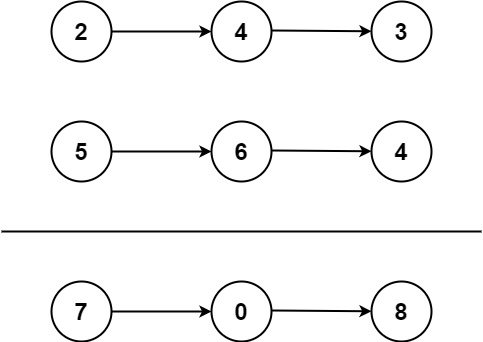# GeetCode Hub

You are given two non-empty linked lists representing two non-negative integers. The digits are stored in reverse order, and each of their nodes contains a single digit. Add the two numbers and return the sum as a linked list.

You may assume the two numbers do not contain any leading zero, except the number 0 itself.

Example 1:```Input: l1 = [2,4,3], l2 = [5,6,4]
Output: [7,0,8]
Explanation: 342 + 465 = 807.
```

Example 2:

```Input: l1 = , l2 = 
Output: 
```

Example 3:

```Input: l1 = [9,9,9,9,9,9,9], l2 = [9,9,9,9]
Output: [8,9,9,9,0,0,0,1]
```

Constraints:

• The number of nodes in each linked list is in the range `[1, 100]`.
• `0 <= Node.val <= 9`
• It is guaranteed that the list represents a number that does not have leading zeros.

/** * Definition for singly-linked list. * public class ListNode { * int val; * ListNode next; * ListNode() {} * ListNode(int val) { this.val = val; } * ListNode(int val, ListNode next) { this.val = val; this.next = next; } * } */ public ListNode addTwoNumbers(ListNode l1, ListNode l2) { return Calculate(l1,l2, 0); } private ListNode Calculate(ListNode l1, ListNode l2, int carry){ var node = new ListNode(); if(l1 == null && l2 == null){ if(carry == 0){ return null; } node.val = carry; node.next = null; } else if(l1 == null){ int sum = l2.val + carry; node.val = sum%10; node.next = Calculate(l1,l2.next,sum/10); } else if(l2 == null){ int sum = l1.val + carry; node.val = sum%10; node.next = Calculate(l1.next,l2,sum/10); } else{ int sum = l1.val + l2.val + carry; node.val = sum%10; node.next = Calculate(l1.next, l2.next, sum/10); } return node; }

We can do this question using both Recursive as well as Iterative approaches.

In the Recursive approach, we will go deep into the recursion until we meet our base condition (i.e both list nodes should be null) and at the same time we will be calculating the node sum and passing the carry to subsequent recursive calls. While doing so, in the end we will get our desired result.

In the Iterative approach, we will be looping through both lists until both become null, and at the same time we will be keeping track of the sum and carry of nodes and keep on adding them in one temp linked list that will contain our result.

Time Complexity: O(max(m,n))  // where m and n are the length of linked list 1 and linked list 2.

Space Complexity: O(max(m,n)+1)  // where m and n are the length of the linked list 1 and linked list 2, and space is for storing the result in a separate linked list.